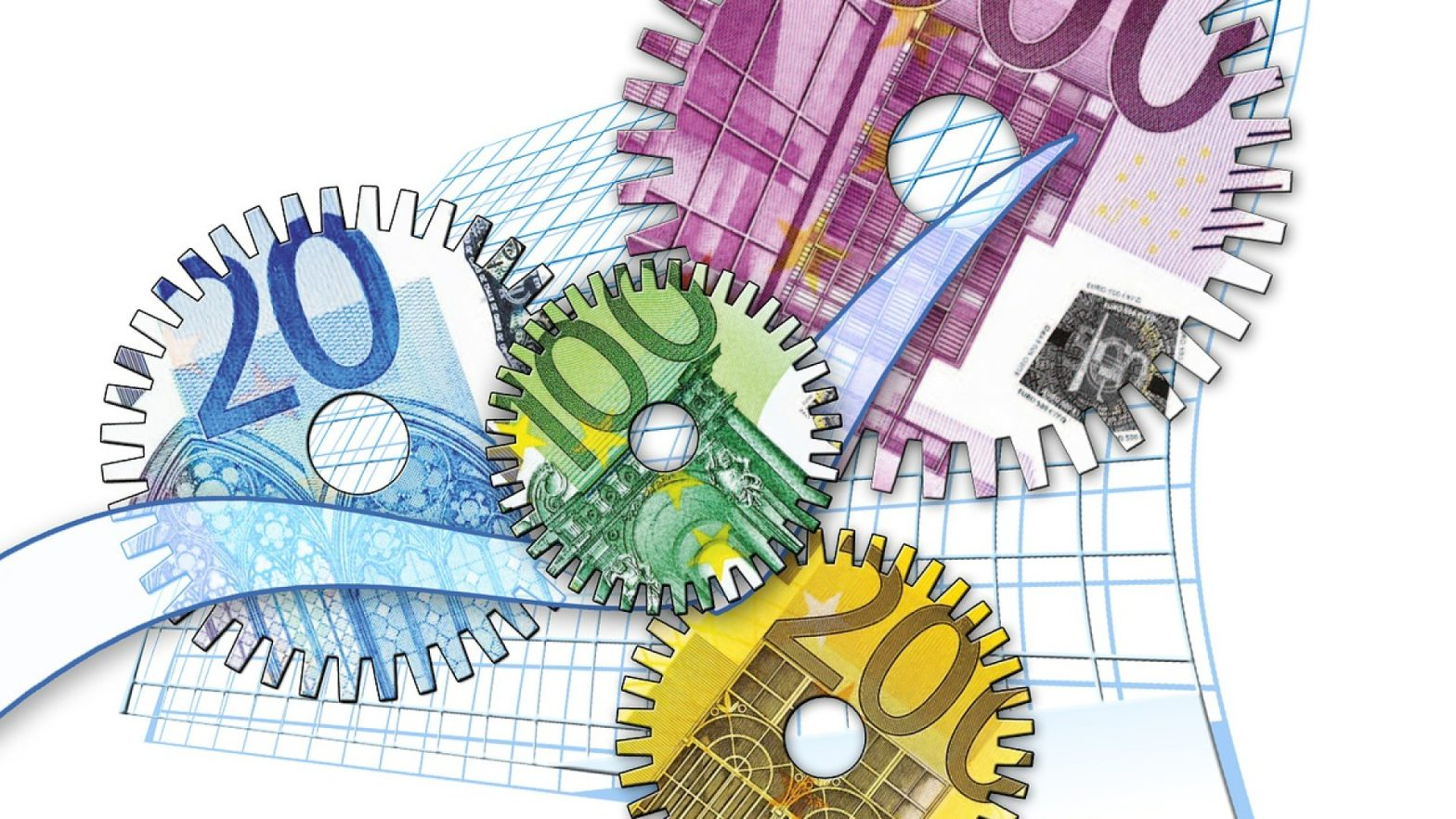# COMPARISON OF SYSTEM BETS AND EXPRESS BETS

## Systems of bigger dimensions

Let’s see what other system sizes are available and what their breakeven thresholds are.

Of particular interest are the four-element systems. There is already a manoeuvre here. It is possible to make a dimension “2-4” or “3-4”.

For the size “2-4” the break-even point would be the coefficient of 1.42. At this variant from four outcomes 6 doubles are formed.

If a parlay were made and all 4 outcomes played, a profit would be made:

300 * 1.42 * 1.42 * 1.42 * 1.42 = 1 220

In the system, \$300 would be divided among six elements, \$50 each If all played, we would get:

6 * (50 * 1.42 * 1.42) = 605

That’s half the profit of the Express.

If one bet loses, 3 doubles lose and 3 win:

3 * (50 * 1.42 * 1.42) = 303

We’re left with ours.

Option “3-4” represents 4 trebles, it already has a break-even point at 1.59. Higher, than in the “2-4”, which is logical.

An express of four events at 1.59 would give a profit:

300 * 1.59 * 1.59 * 1.59 * 1.59 = 1 917

A fully winning 3-4 system would give:

4 * (75 * 1.59 * 1.59 * 1.59) = 1 206

For the sake of comparison let’s calculate the system with the size “2-4”, but with the odds 1.59.

If all 4 bets are played, we get:

6 * (50 * 1.59 * 1.59) = 758

If one loses, and 3 win:

3 * (50 * 1.59 * 1.59) = 379

As you can see the total winning profit here is almost 2.5 times lower than in the Express, and by 37% in comparison with the winning systems of size “3-4”. But there is an option for winning just two positions out of four. In this case both the Express and the 3-4 will be lost.

50 * 1.59 * 1.59 = 126

That is not all the money will be lost, but about 42% of the played money will be saved. This can be perceived as some kind of additional bonus.

## Ratio of risks and profitability of expresses and systems

When multiplying the odds and risks are also multiplied. Here we take the ideal case where the bookmaker’s quotes reflect the real probability minus the margin. This, of course, is not always the case. The bettor’s task is to avoid outcomes that are clearly undervalued or loaded with a bunch of “pop-ups”. It is necessary to take only adequately priced events, or with the presence of a certain preponderance in favor of the player. But these are nuances from the topic of other articles. Here we will assume that odds are adequate and margin is equal. The task is to compare the probability of winning the express and the system. By comparing the size of these winnings, we will be able to make important conclusions about the appropriateness of these designs.

For comparison, let’s take the same variants we started with: a treble and a “2-3” system with quotes of 1.74.

Let’s assume that the pair of outcomes is priced as: 1.74 to 2.21. The margin is 2.72%. At a leverage of 1.74 it would be 1.52%. The real probability without margin would be 55.95%, or as a fraction: 0.5595. To find out the probability of winning the express, multiply the probabilities:

0.5595 3 = 0.175

Or 17.5%.

The probability of winning the whole system is identical, because all three pluses are needed.

The probability of winning a double in the system and getting a return:

0.5595 2 = 0.313

Or 31.3%.

Let’s compare the potential profit and probabilities. Let’s take a distance of 3000 bets. From these, 1000 combinations of 3 will be formed, which will create the expresses and systems. Given the probability, only 175 out of the 1000 expresses will win.

Let’s assume we still bet \$300 on each Parlay. This would represent a total of \$300,000

175 * (300 * 1.74 * 1.74 * 1.74) = 276 571

The loss would be:

276 571 – 300 000 = -23 429

The reason is the margin contained in each quote. Due to the multiplication within an express, this drawdown is obtained. Let’s take a look at the systems.

Given the calculated probabilities, at the same distance 175 systems will also win and another 313 will give a return.

175 * [ 3 * (1.74 * 1.74 * 100) ] = 158 949

313 * (1.74 * 1.74 * 100) = 94 784

158 949 + 94 784 = 253 733

The loss will be:

253 733 – 300 000 = -46 267

We have clearly proved that expresses are more profitable than systems over the distance. Curtain.